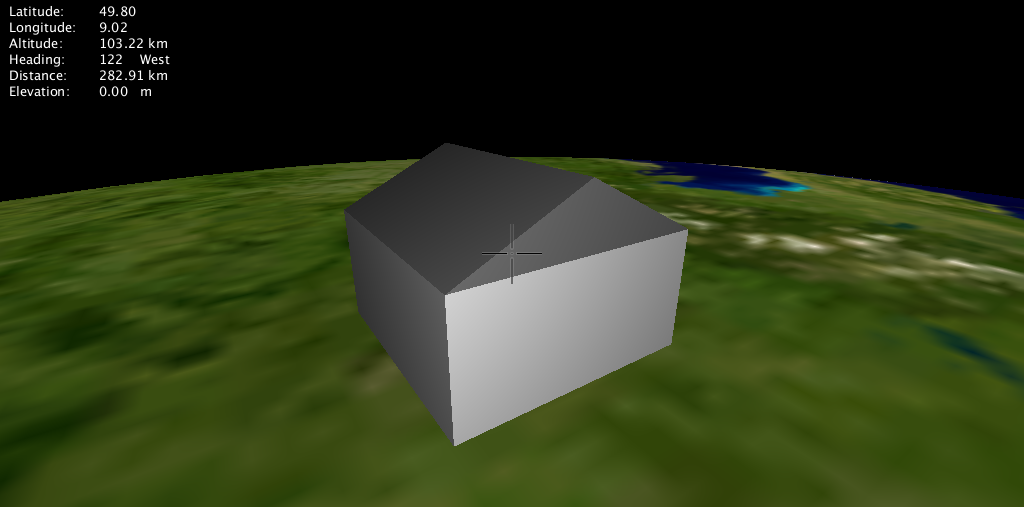LibMini

## Programming Example

In this programming example we are going to put a building on a place on earth.

The place on earth is defined as a local coordinate system at a geo-referenced position. Possible geographic reference systems are Lat/Lon, UTM, Mercator or ECEF. The z-axis of the local coordinate system points upwards and the y-axis points north. The units of the local coordinate system are meters.

The building is modeled in the local coordinate system as a scene graph that contains two simple geometric objects, a cube as the base and a prism as the roof.

The scene graph is constructed from a set of graph nodes as follows:

• derive from Viewer class
• overwrite build_ecef_geometry method to describe the scene as a graph
• construct scene graph to contain a single house in a local coordinate system
• at position Lat/Lon = 49 degrees latitude, 11 degrees longitude
• via appending a coord node to the scene graph
• and define the local scale of the building
• via appending a scale node
• and define the appearance of the building’s components
• via appending color nodes
• and define the proper positions of the components in the local coordinate system
• and add the geometry of the house to the scene graph
• via appending cube and prism nodes

All graph nodes are derived from libMini’s mininode base class.

class Renderer: public Viewer
{
protected:

mininode_group *Renderer::build_ecef_geometry()
{
// define local coordinate system at Lat/Lon=49/11
mininode *house = new mininode_coord(minicoord(miniv3d(11*3600, 49*3600, 0),
minicoord::MINICOORD_LLH));

// scale up by a factor of 10000
mininode *scale = house->append_child(new mininode_scale(10000));

// white cube with base size 10x10 and height 5 meters
// origin of the cube is its barycenter
// translate up 2.5 meters
scale->append_child(new mininode_color(miniv3d(1.0, 1.0, 1.0)))->
append_child(new mininode_translate(miniv3d(0.0, 0.0, 2.5)))->
append_child(new mininode_geometry_cube(10.0, 10.0, 5.0));

// grey prism as roof with base size 10x10
// origin of the prism is the center of its base plane
// translate up 5 meters
scale->append_child(new mininode_color(miniv3d(0.5, 0.5, 0.5)))->
append_child(new mininode_translate(miniv3d(0.0, 0.0, 5.0)))->
append_child(new mininode_geometry_prism(10.0, 10.0, 2.5));

return(house);
}
};

The house as it looks like in the QTViewer: